# Image Mosaics With Python, Part II

### 15 January 2017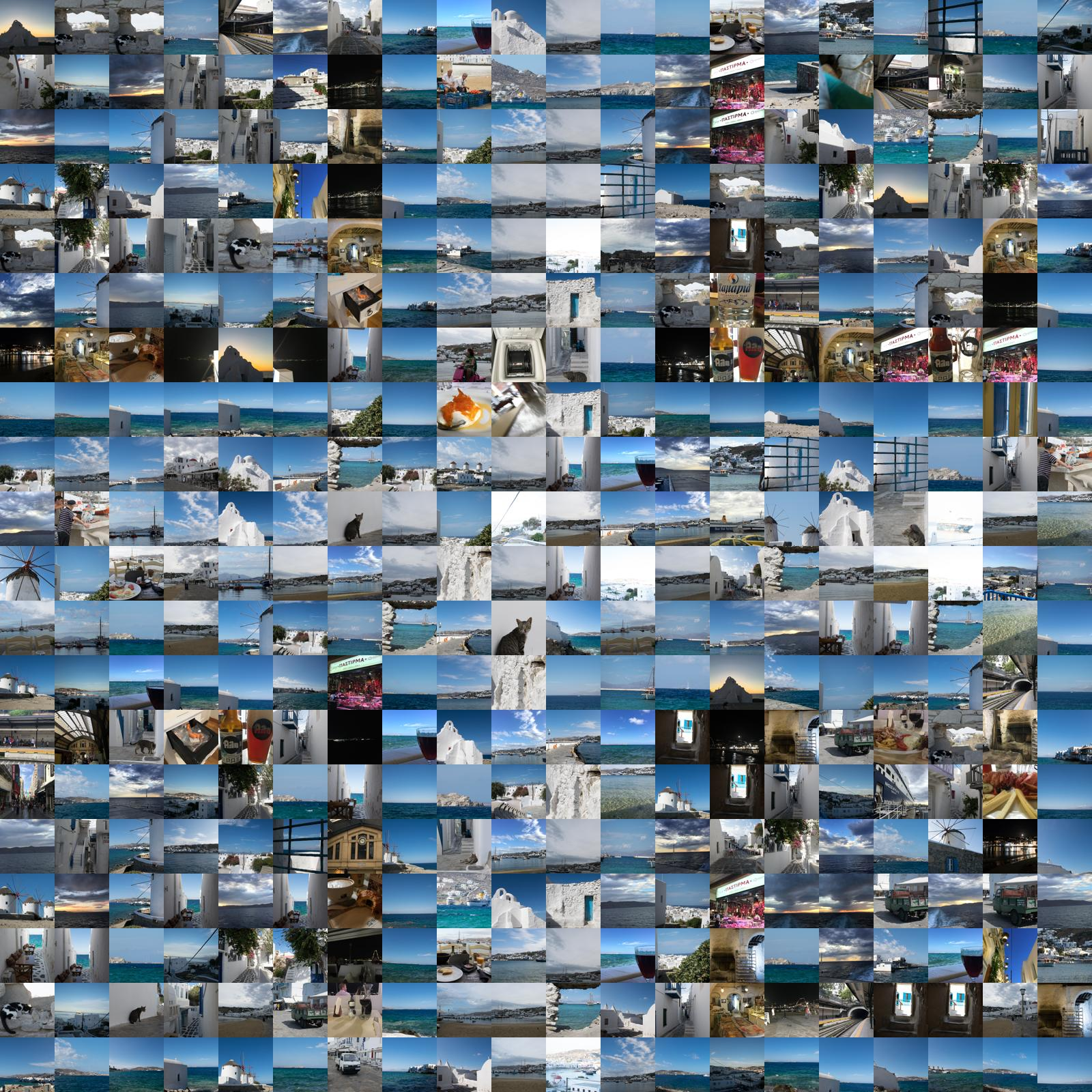In the second part of the series on image mosaics, we explore creating mosaics with a stochastic method.

## What do you mean by stochastic?

By stochastic, I mean that the output image is constructed by a series of random actions.

The first of these is selecting a set of tile images. We choose an image from our pool of source images to represent each pixel in the reference images. At this point, the image is completely random.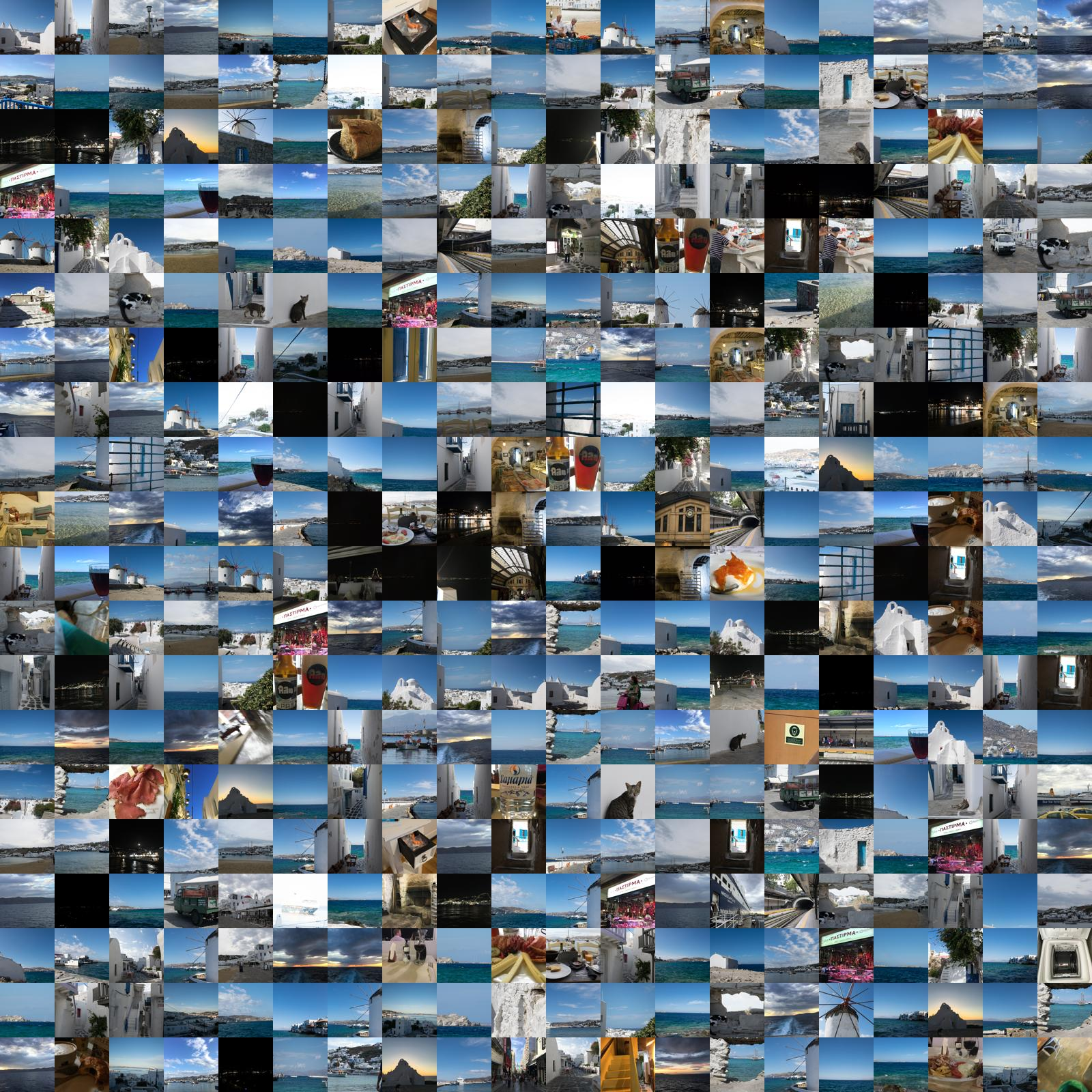We then select pairs of pixels in the reference image at random. Examining the current source images assigned to each of these reference pixels, we see if the output image would be improved by swapping them. If so, we swap the images.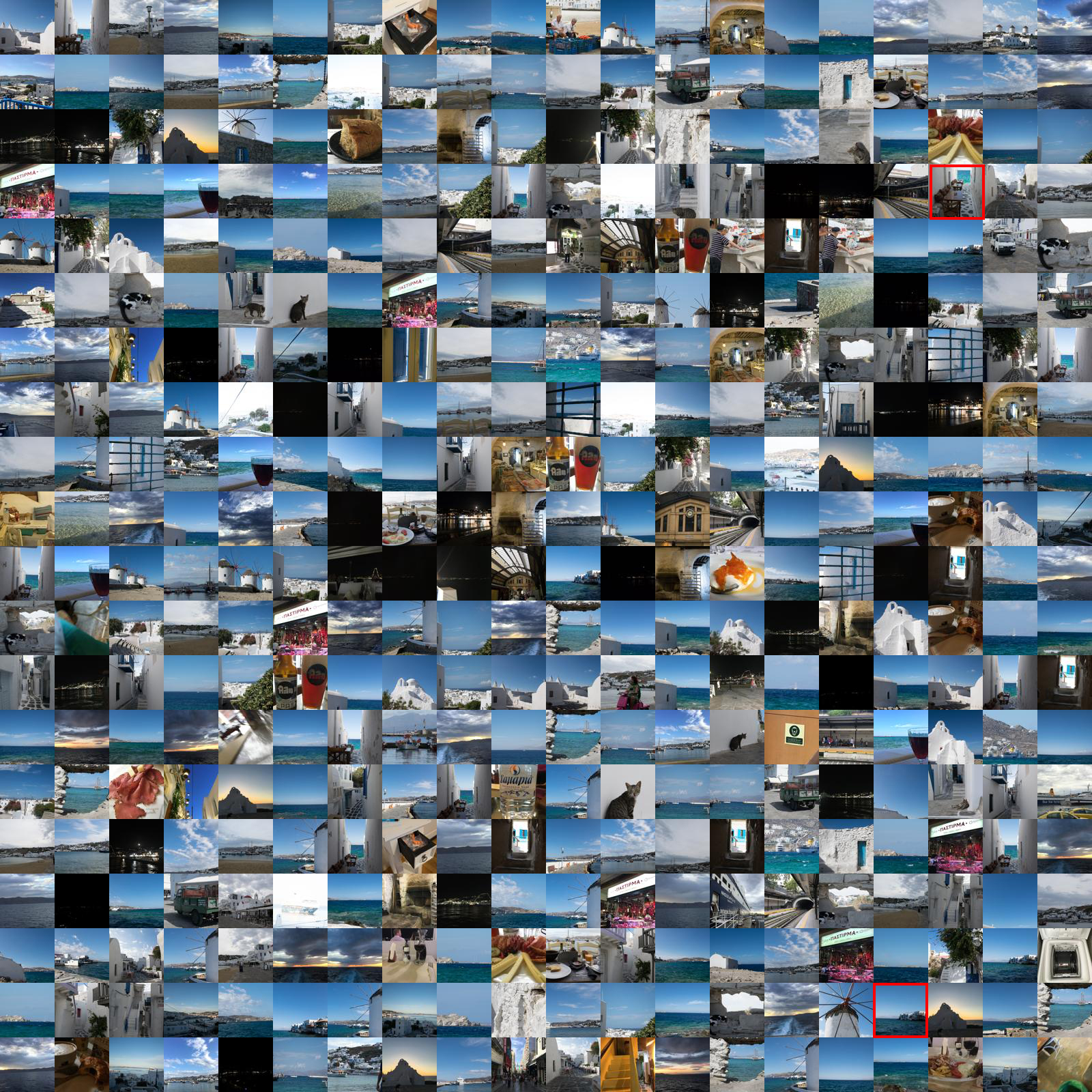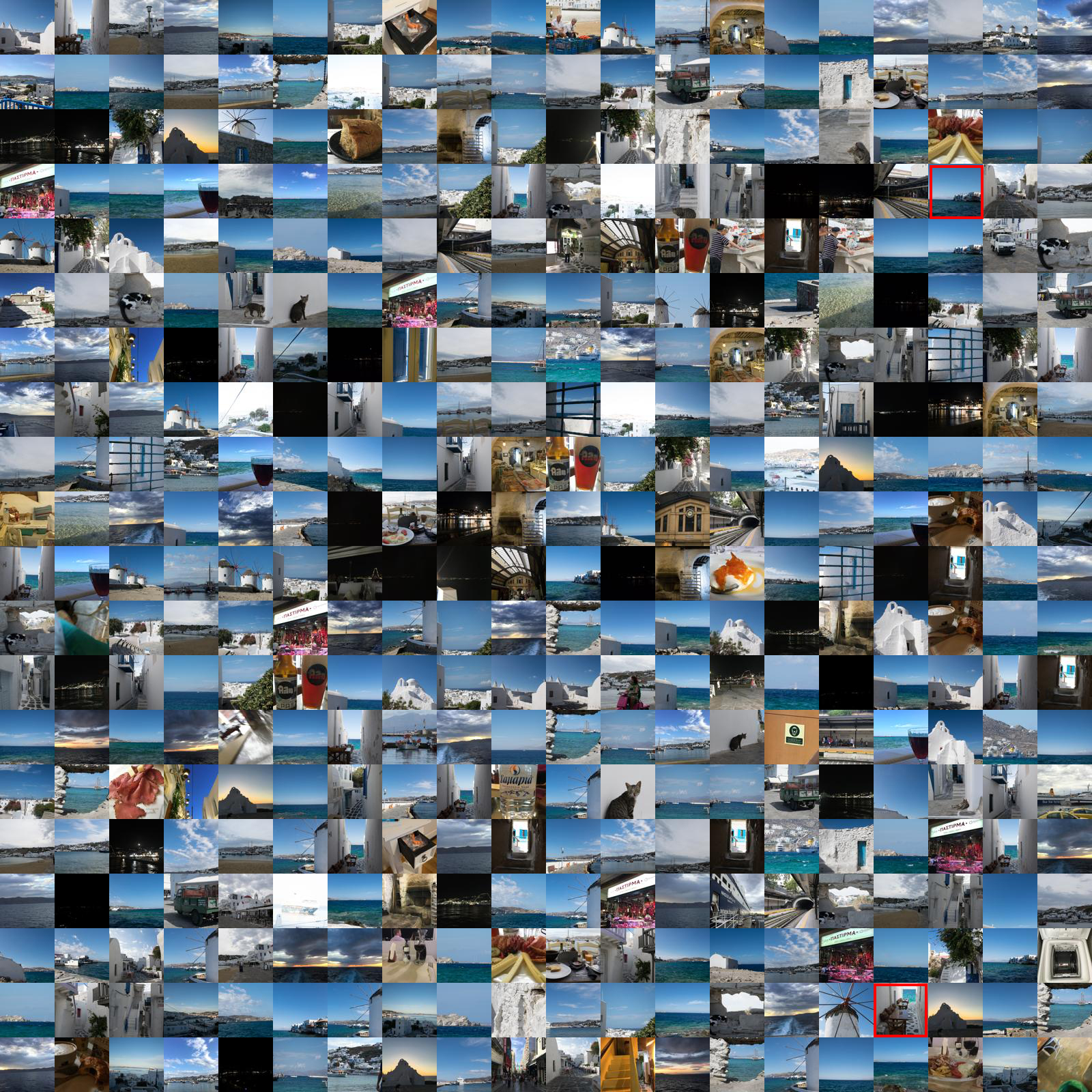We then perform this operation thousands of times. At the end we (hopefully) have an mosaic image that looks like the reference image.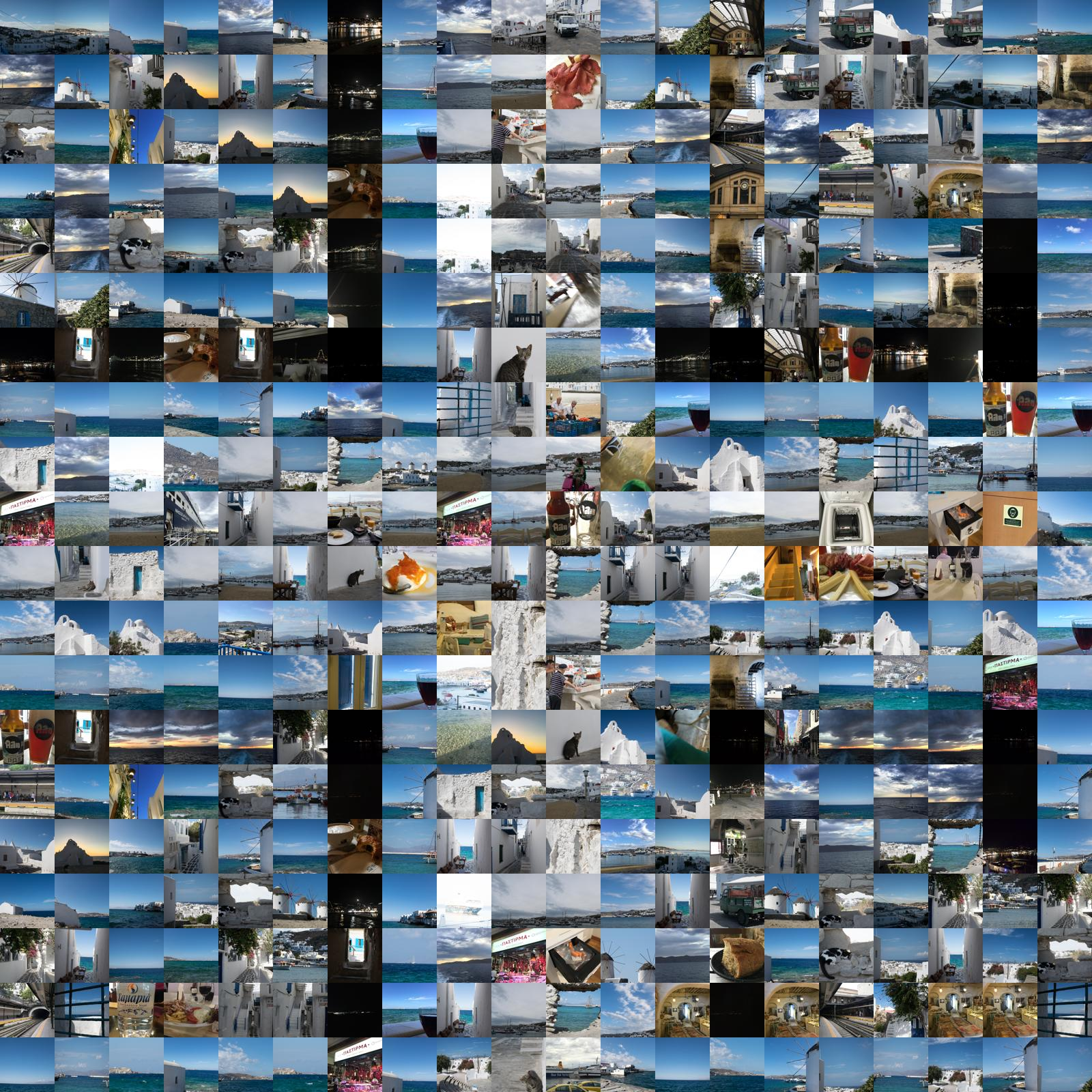## When do we swap?

In the first installment of this series, we defined how well a pixel in the reference image was represented by a source image by calculating a representative color from the source image and comparing it to the reference pixel's color. This comparison was performed using the norm function, which, essentially, calculates the distances between the two colors by treating the RGB values as XYZ coordinates.

Using this same distance logic, we decide to swap whenever the distance represented by the swap is less than the distance represented by the current arrangement:

```# swapping is an improvement, perform the swap
if swapped_norm_1 + swapped_norm_2 < current_norm_1 + current_norm_2:

output_matrix[loc_1][loc_1] = source_2
output_matrix[loc_2][loc_2] = source_1```

By performing this operation thousands of times, we expect to reduce the overall "distance" between the reference image and output mosaic.

## Initial population influence

In generating a mosaic in this way, the initial population of images has more influence on the output image than in the methods mentioned in the first part. This is because if the pool of available images is larger than the number needed to generate the output mosaic we potentially don't have access to better matches if we start by selecting a random subset of the pool.

Of course, if the output mosaic is larger than the pool, there is still some impact. This is because images will be repeated, but the repeated images may not be the "best" ones.

There are some solutions to this problem. One possible approach is randomly choose an image from the pool to swap with an image currently selected to generate the output mosaic. We could set a threshold of, say, 0.1, and write the appropriate code to say "if `random.random() < 0.1` swap from the pool". This approach is not implemented, but should be straightforward.

As always, there is value in running the algorithm multiple times and attempting to select an output that looks good visually.

## Talking 'bout my generation(s)

The default number of generations is 1,000,000. This is overkill for smaller images, but works better for larger ones.

We can actually watch the image converge by generating an output image for each generation and then stitching them into an animation (first 10,000 generations):

## Do you have this code somewhere that I can use it?

Funny you should ask! Yes: GitHub. See here for this part specifically.